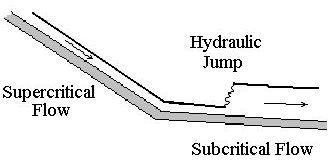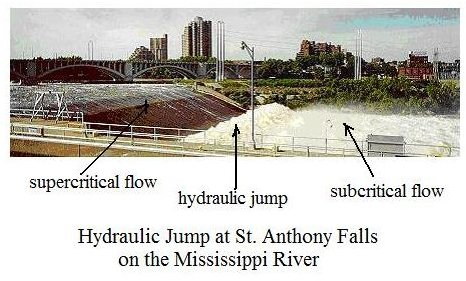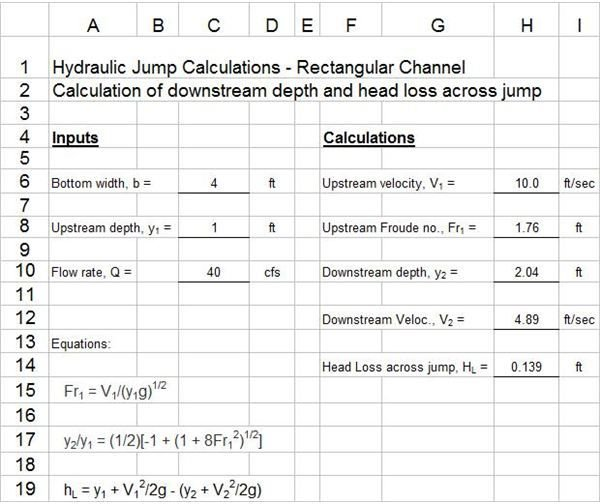# Excel Formulas for Hydraulic Jump Calculations in a downloadable Excel Spreadsheet Templates

## Background on the Hydraulic Jump in Open Channel Flow

A hydraulic jump will occur whenever there is supercritical flow taking place in an open channel with a subcritical slope. The hydraulic jump provides anabrupt adjustment from supercritical to subcritical flow. This is necessary because the subcritical slope cannot sustain a supercritical flow. The diagram at the right shows a physical open channel flow situation that would give rise to a hydraulic jump. For more background on hydraulic jumps see the article, "Open Channel Flow Basics – Hydraulic Jump Calculations." For additional information on subcritical and supercritical open channel flow, see the article, "Open Channel Flow Basics 2 – Supercritical Flow." For an Excel spreadsheet template that can be downloaded to do hydraulic jump calculations, read on in this article.

## Equations for Hydraulic Jump Calculations

For flow in a rectangular open channel, with known channel width (b), upstream (supercritical) depth of flow (y1), and volumetric flow rate (Q), thedownstream (subcritical) depth of flow (y2) can be calculated from the following equations:

y2/y1 = (1/2)[-1 + (1 + 8Fr12)1/2] ; V1 = Q/(by1) ; and Fr1 = V1/(y1g)1/2.

The upstream velocity, V1 and upstream Froude number, Fr1, need to be calculated first, using the last two equations, then the downstream depth of flow, y2 can be calculated using the first equation. After y2 is calculated, the downstream velocity of flow, V2, can be calculated from V2 = Q/(by2).

There will always be frictional head loss across a hydraulic jump, due to the turbulence in the jump. That frictional head loss, hL, can be calculated from the equation:

hL = y1 + V12/2g – (y2 + V22/2g).

An Excel spreadsheet template for making the above open channel flow calculations is presented and discussed in the next section.

## Hydraulic Jump Calculations Using Excel FormulasExcel spreadsheet templates can be used to easily make hydraulic jump calculations for open channel flow with the equations discussed above. Once the Excel formulas are entered, you simply need to enter the required input values and the Excel spreadsheet will make the calculations. The Excel template at the left is set up the make these calculations.

This Excel spreadsheet template requires input values for the upstream depth of flow, y1, the channel width, b, and the volumetric flow rate through the channel, Q. Then the Excel formulas that have been entered into the spreadsheet template will calculate the upstream velocity, V1, the upstream Froude number, Fr1, the downstream depth of flow, y2, and the downstream velocity, V2.

## References and Image Credits

References for further information:

1. Bengtson, Harlan H. Open Channel Flow II – Hydraulic Jumps and Supercritical and Nonuniform FlowAn online, continuing education course for PDH credit.

2. Munson, B. R., Young, D. F., & Okiishi, T. H., Fundamentals of Fluid Mechanics, 4th Ed., New York: John Wiley and Sons, Inc, 2002.

3. Chow, V. T., Open Channel Hydraulics, New York: McGraw-Hill, 1959.

Image Credits:

1. Wikipedia Commons, StAnthonyFalls apron

2. Hydraulic Jump Diagram – drawn by H. Bengtson

## This post is part of the series: Open Channel Flow Basics

Open channel flow takes place with a free surface open to the atmosphere, so flow is due to gravity, rather than due to pressure as in pipe flow. The Manning equation applies for uniform flow. A hydraulic jump is nonuniform flow. It occurs for supercritical flow on a subcritical channel slope.